Force And Laws of Motion - NCERT Questions
Q 1.

Which of the following has more inertia:
(A) a rubber ball and a stone of the same size?
(B) a bicycle and a train ?
(C) a five-rupees coin and a one-rupee coin?

SOLUTION:

(A) A stone of the same size as rubber ball has more mass. As mass is a measure of inertia, therefore, stone has more inertia than a rubber ball.
(B) A train has greater inertia than a bicycle, because train is much heavier than a bicycle.
(C) A five-rupees coin is heavier than a one-rupee coin. Therefore, inertia of five-rupees coin is more than the inertia of one-rupee coin.

Q 2.

In the following example, try to identify the number of times the velocity of the ball changes:
“A football player kicks a football to another player of his team who kicks the football towards the goal. The goalkeeper of the opposite team collects the football and kicks it towards a player of his own team”.
Also identify the agent supplying the force in each case.

SOLUTION:

(i) Velocity of the football changes when first player kicks the ball towards another player of his team.
(ii) Velocity of the football also changes when another player kicks the football towards the goal.
(iii) Velocity of the football also changes when the goalkeeper of the opposite team stops the football by collecting it.
(iv) Velocity of the football changes when the goalkeeper kicks it towards a player of his team.
Thus, the velocity of the ball changes four times in this case.
In first and second cases, the force is supplied by the foot of players. In third case, force is supplied by the hands of the goalkeeper. In fourth case, as the goalkeeper hits the football with his foot, so the foot of the goalkeeper supplies the force.

Q 3.

Explain why some of the leaves may get detached from a tree if we vigorously shake its branch.

SOLUTION:

When a tree is vigorously shaken, the branches of the tree come in motion but the leaves tend to continue in their state of rest due to inertia of rest. As a result of this, leaves get separated from the branches of the tree and hence fall down.

Q 4.

Why do you fall in the forward direction when a moving bus brakes to a stop and fall backwards when it accelerates from rest?

SOLUTION:

When a moving bus brakes to a stop, our feet come to rest with the bus. But upper part of our body continues to move forward on account of inertia of motion. That is why we tend to fall in the forward direction.
However, when the bus accelerates from rest, lower part of our body moves with the bus. The upper part of the body tries to maintain itself at rest on account of inertia of rest. Therefore, we tend to fall backwards.

Q 5.

If action is always equal to the reaction, explain how a horse can pull a cart.

SOLUTION:

It is true that when a horse pulls a cart with a certain force, then the cart also pulls the horse with the same force and the system of horse and cart should not move.
However, if we analyse the problem carefully, then horse not only applies force on the cart, but also pushes the earth backward with his feet. In this action of the horse, the earth reacts back and pushes it in the forward direction. Now, the force applied by the horse on the earth is insufficient to move the earth, but the force applied by the earth is sufficient to make the horse to move in the forward direction.
It is this unbalanced force applied by the earth, which makes the system of horse and cart to move in the forward direction.

Q 6.

Explain, why is it difficult for a fireman to hold a hose, which ejects large amount of water at a high velocity ?

SOLUTION:

The ejection of a large amount of water at a high velocity from a hosepipe results in the development of an equal force of reaction on the hosepipe in the backward direction. That is why it becomes difficult for the fireman to hold the hosepipe.

Q 7.

From a rifle of mass 4 kg, a bullet of mass 50 g is fired with an initial velocity of 35 m s–1. Calculate the initial recoil velocity of the rifle.

SOLUTION:

Initial mass of rifle m1 = 4 kg
Let initial velocity of recoil of rifle = v1
∴ Initial momentum of the rifle = 4 kg × v1
Initial mass of bullet m2= 50 g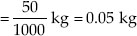Initial velocity of bullet v2 = 35 m s–1
∴ Initial momentum of bullet
= 0.05 kg × 35 m s–1
By the law of conservation of momentum,
Momentum of the rifle = Momentum of bullet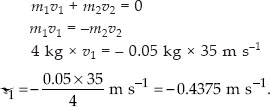Negative sign indicates that rifle moves in a direction opposite to the direction of bullet.

Q 8.

Two objects of masses 100 g and 200 g are moving along the same line and direction with velocities of 2 m s–1 and 1 m s–1 respectively. They collide and after the collision, the first object moves at a velocity of 1.67 m s–1. Determine the velocity of second object.

SOLUTION: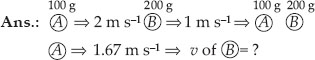Let the 100 g and 200 g objects be A and B as shown in diagram,
∴ Initial momentum of A = 100 g × 2 m s–1
= 200 g m s–1.
Initial momentum of B = 200 g × 1 m s–1
= 200 g m s–1
∴ Total momentum of A and B before collision
= (200 + 200) g m s–1 = 400 g m s–1
Let the velocity of B after collision = v
∴ Momentum of B after collision = 200 g × v
Also, momentum of A after collision
= 100 g × 1.67 m s–1 = 167 g m s–1
∴ Total momentum of A and B after collision = 200 g × v + 167 g m s–1
Now, by the law of conservation of momentum,
Momentum of A and B after collision
= Momentum of A and B before collision
200 g × v + 167 g m s–1 = 400 g m s–1
200 g × v = (400 – 167) g m s–1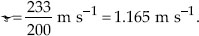Q 9.

An object experiences a net zero external unbalanced force. Is it possible for the object to be travelling with a non-zero velocity? If yes, state the conditions that must be placed on the magnitude and direction of the velocity. If no, provide a reason.

SOLUTION:

Yes, when external unbalanced force on an object is zero, the object can be travelling with a non-zero velocity. The necessary conditions are :
(i) The object should already be moving with a uniform speed along a straight line.
(ii) There should be no change in magnitude of velocity and also no change in the direction of motion.
(iii) The resistance to motion due to air must be zero.
(iv) The resistance to motion due to friction between the object and the ground must be zero.
If any of the four conditions stated above is not met with, the answer will be no. This is because external unbalanced force is needed for initiating the motion and also for any subsequent change (decrease/increase) in its velocity.

Q 10.

When a carpet is beaten with a stick, dust comes out of it. Explain..

SOLUTION:

Initially, both carpet and the dust herein are at rest. When the carpet is beaten with a stick, the carpet is set into motion. Due to inertia of rest, the dust particles tend to remain at rest. As a result, the dust particles fall off.

Q 11.

Why is it advised to tie any luggage kept on the roof of a bus with a rope ?

SOLUTION:

A luggage is usually tied with a rope on the roof of buses. When a moving bus suddenly stops, the luggage on its roof tends to continue in the state of motion due to inertia of motion. Hence the luggage fall down from the roof of the bus. Similarly, when a bus suddenly starts, the luggage on the roof of the bus tends to continue in the state of rest and hence fall down from the roof of the bus. Thus, to avoid the falling of the luggage, it is tied with a rope on the roof of a bus.

Q 12.

A batsman hits a cricket ball which then rolls on a level ground. After covering a short distance, the ball comes to rest. The ball slows to a stop because
(A) the batsman did not hit the ball hard enough.
(B) velocity is proportional to the force exerted on the ball.
(C) there is a force on the ball opposing the motion.
(D) there is no unbalanced force on the ball, so the ball would want to come to rest.

SOLUTION:

The cricket ball comes to rest after covering a short distance, because there is a force on the ball, opposing the motion. This force is due to resistance of air and also due to friction between the ball and the ground.

Q 13.

A truck starts from rest and rolls down a hill with a constant acceleration. It travels a distance of 400 m in 20 s. Find its acceleration. Find the force acting on it if its mass is 7 tonnes.
(Hint : 1 tonne = 1000 kg.)

SOLUTION:

Due to constant movement of particles colloidal particles do not settle down.

Q 14.

A stone of 1 kg is thrown with a velocity of 20 m s–1 across the frozen surface of a lake and comes to rest after travelling a distance of 50 m. What is the force of friction between the stone and the ice?

SOLUTION: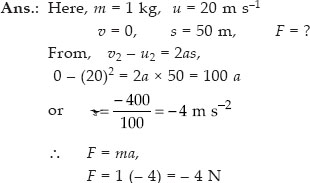Negative sign indicates that force of friction is opposing the motion of the ball.

Q 15.

A 8000 kg engine pulls a train of 5 wagons, each of 2000 kg, along a horizontal track. If the engine exerts a force of 40000 N and the track offers a friction force of 5000 N, then calculate
(A) the net accelerating force;
(B) the acceleration of the train; and
(C) the force of wagon 1 on wagon 2

SOLUTION:

Net accelerating force
= Force exerted by engine – Frictional force
= 40000 – 5000 = 35000 N.
(B) Acceleration,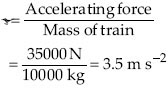(C) Force of wagon 1 on wagon 2
= 8000 kg × 3.5 m s–2 = 28000 N.

Q 16.

An automobile vehicle has a mass of 1500 kg. What must be the force between the vehicle and road if the vehicle is to be stopped with a negative acceleration of 1.7 m s–2?

SOLUTION:

Here, m = 1500 kg, a = – 1.7 m s–2, F = ?
F = ma
F = 1500 (– 1.7) N = – 2550 N
Negative sign indicates that force is opposing the motion of the vehicle.

Q 17.

What is the momentum of an object of mass m, moving with a velocity v ?
(A) (mv) 2
(B) mv 2
(C)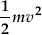(D) mv

SOLUTION:

Momentum = mass × velocity
= m × v = mv

Q 18.

Using a horizontal force of 200 N, we intend to move a wooden cabinet across a floor at a constant velocity. What is the friction force that will be exerted on the cabinet ?

SOLUTION:

The cabinet will move with constant velocity, if net external force acting on it is zero. Since a horizontal force of 200 N acts on the cabinet in the forward direction, therefore, net external force acting on it will be zero if frictional force of 200 N acts on it. Thus frictional force = 200 N will be exerted on the cabinet.

Q 19.

Two objects, each of mass 1.5 kg, are moving in the same straight line but in opposite directions. The velocity of each object is 2.5 m s–1 before the collision during which they stick together. What will be the velocity of the combined object after collision ?

SOLUTION:

Let the two objects are A and B.
Mass of object A, m1 = 1.5 kg
Mass of object B, m2 = 1.5 kg
Velocity of object A before collision,
u1 = 2.5 m s–1
Velocity of object B before collision,
u2 = – 2.5 m s–1
∴ Total momentum of object A and B before collision = m1u1 + m2u2
= 1.5 × 2.5 – 1.5 × 2.5 = 0
Mass of combined object after collision
= (m1 + m2) = 3.0 kg
Let, velocity of combined object after collision = V m s–1
∴ Total momentum of combined object after collision = (m1 + m2)V = (3V) kg m s–1
According to the law of conservation of momentum
Momentum after collision = Momentum before collision
i.e. 3V = 0 or V = 0

Q 20.

According to the third law of motion when we push an object, the object pushes back on us with an equal and opposite force. If the object is a massive truck parked along the roadside, it will probably not move. A student justifies this by answering that the two opposite and equal forces cancel each other. Comment on this logic and explain why the truck does not move.

SOLUTION:

Student’s justification is not correct. Two equal and opposite forces cancel each other if they act on the same body. According to the third law of motion, action and reaction forces are equal and opposite but they both act on different bodies. Hence, they cannot cancel each other.
When we push a massive truck, then the force applied on the truck is not sufficient to overcome the force of friction between the tyres of the truck and ground. Hence the truck does not move. The truck will move only if the force applied on it is greater than the frictional force.

Q 21.

A hockey ball of mass 200 g travelling at 10 m s–1 is struck by a hockey stick so as to return it along its original path with a velocity at 5 m s–1. Calculate the change of momentum occurred in the motion of the hockey ball by the force applied by the hockey stick.

SOLUTION:

Mass of ball (m) = 200 g = 0.2 kg
Initial velocity of ball (u1) = 10 m s–1
∴ Initial momentum of ball = mu1
= 0.2 kg × 10 m s–1 = 2 N s
Final velocity of ball (u2) = – 5 m s–1
(Minus sign denotes that ball is moving in opposite direction.)
∴ Final momentum of ball = mu2
= 0.2 kg × – 5 m s–1 = – 1 N s
∴ Change in momentum
= Final momentum – Initial momentum
= – 1 N s – 2 N s = – 3 N s.
Minus sign denotes that change in momentum is in the direction opposite to the direction of initial momentum of the ball.

Q 22.

A bullet of mass 10 g travelling horizontally with a velocity of 150 m s–1 strikes a stationary wooden block and comes to rest in 0.03 s. Calculate the distance of penetration of the bullet into the block. Also calculate the magnitude of the force exerted by the wooden block on the bullet.

SOLUTION:

Mass of bullet (m) = 10 g = 0.01 kg
Initial velocity of bullet (u) = 150 m s–1
Final velocity of bullet (v) = 0
Time (t) = 0.03 s.
Acceleration on bullet (a) = ?
Distance penetrated by bullet (s) = ?
Force acting on wooden block (F) = ?
(i) Applying , v = u + at.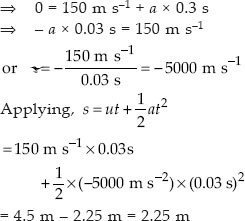(ii) Applying, F = ma
Force acting on bullet (F)
= 0.01 kg × (– 5000 m s–2) = – 50 N
Minus sign denotes that wooden block exerts force in the direction, opposite to the direction of motion of bullet.

Q 23.

An object of mass 1 kg travelling in a straight line with a velocity of 10 m s–1 collides with, and sticks to, a stationary wooden block of mass 5 kg. Then they both move off together in the same straight line. Calculate the total momentum just before the impact and just after the impact. Also, calculate the velocity of the combined object.

SOLUTION:

Mass of object, m1 = 1 kg
Velocity of object before collision,
u1 = 10 m s–1
Mass of wooden block, m2 = 5 kg
Velocity of wooden block before collision, u2 = 0
(i) ∴ Total momentum before the impact
= m1u1 + m2u2 = 1 × 10 + 5 × 0
= 10 kg m s–1
According to the law of conservation of momentum (as no net external force acts on the system) :
Total momentum after the impact = Total momentum before the impact = 10 kg m s–1
(ii) Mass of combined object, M = mass of object + mass of block = 1 + 5 = 6 kg
Let, V = Velocity of the combined object after collision
∴ Momentum of combined object
= MV
= (6V) kg m s–1
Now, momentum of combined object = Total momentum after the impact
i.e. 6V = 10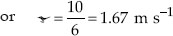Q 24.

An object of mass 100 kg is accelerated uniformly from a velocity of 5 m s–1 to 8 m s–1 in 6 s. Calculate the initial and final momentum of the object. Also, find the magnitude of the force exerted on the object.

SOLUTION:

Here, m = 100 kg
u = 5 m s–1
v = 8 m s–1, t = 6 s
Initial momentum of object, p1 = mu
= 100 × 5 = 500 kg m s–1
Final momentum of object, p2 = mv
= 100 × 8 = 800 kg m s–1

Q 25.

Akhtar, Kiran and Rahul were riding in a motorcar that was moving with a high velocity on an expressway when an insect hit the windshield and got stuck on the windscreen. Akhtar and Kiran started pondering over the situation. Kiran suggested that the insect suffered a greater change in momentum as compared to the change in momentum of the motorcar (because the change in the velocity of the insect was much more than that of the motorcar). Akhtar said that since the motorcar was moving with a larger velocity, it exerted a larger force on the insect. And as a result the insect died. Rahul while putting an entirely new explanation said that both the motorcar and the insect experienced the same force and a change in their momentum. Comment on these suggestions.

SOLUTION:

Kiran’s suggestion is incorrect as momentum is always conserved, i.e., change in momentum of insect must be equal to that of motorcar.
Akhtar’s suggestion is incorrect for the same reason as above.
Rahul’s suggestion is correct, i.e., insect and motor-car experience same force and change in momentum. However, the insect died because, it was unable to bear the large force and large change in momentum.

Q 26.

How much momentum will a dump-bell of mass 10 kg transfer to the floor if it falls from a height of 80 cm ? Take its downward acceleration to be 10 m s–2

SOLUTION:

Here, m = 10 kg, s = 80 cm = 0.8 m, a = 10 m s–2
u = 0, v = ?, p = ?
From, v2u2 = 2as, v2 – 0 = 2 × 10 × 0.8 = 16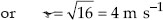Momentum transferred, p = mv = 10 × 4
= 40 kg m s–1

Q 27.

The following is the distance-time table of an object in motion :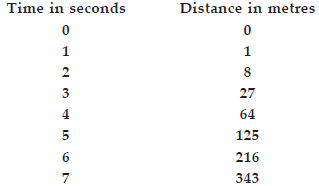SOLUTION:

(A) Here u = 0
Using s = ut + 1/2 at2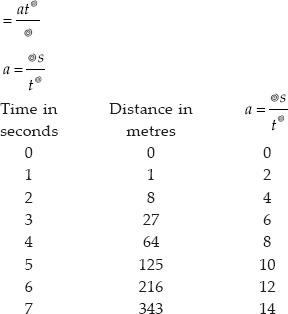Thus acceleration is increasing.
(B) As F = ma, therefore, F µ a. Hence, the force must also be increasing uniformly with time.

Q 28.

Two persons manage to push a motorcar of mass 1200 kg at a uniform velocity along a level road. The same motorcar can be pushed by three persons to produce an acceleration of 0.2 m s–2. With what force does each person push the motorcar ? (Assume that all persons push the motorcar with the same muscular effort.)

SOLUTION:

Here, mass of motorcar, m = 1200 kg
Let each person exert a push F on the motorcar.
Total push of two persons = F + F = 2 F
As this push gives a uniform velocity to the motocar along a level road, it must be a measure of the force of friction (f) between the motorcar and the road, i.e., f = 2F.
When three person push, total force applied = F + F + F = 3 F
Force that produces acceleration (a = 0.2 m s–2), i.e., ma = 3 Ff = 3F – 2 F = F
or F = ma = 1200 × 0.2 = 240 N

Q 29.

A hammer of mass 500 g, moving at 50 m s–1, strikes a nail. The nail stops the hammer in a very short time of 0.01 s. What is the force of the nail on the hammer ?

SOLUTION:

The force of nail on the hammer,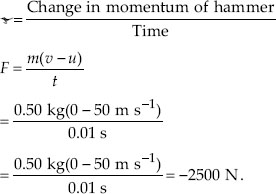Minus sign denotes the force of nail on the hammer, is acting in the direction, opposite to that of hammer.

Q 30.

A motorcar of mass 1200 kg is moving along a straight line with a uniform velocity of 90 km/h. Its velocity is slowed down to 18 km/h in 4 s by an unbalanced external force. Calculate the acceleration and change in momentum. Also calculate the magnitude of the force required.

SOLUTION:

Here, mass, m = 1200 kg
Initial velocity, u = 90 km h–1
Final velocity, v = 18 km h–1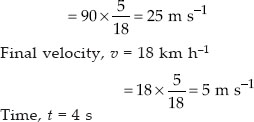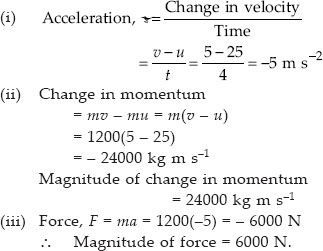Q 31.

A large truck and a car, both moving with a velocity of magnitude v, have a head-on collision and both of them come to a halt after that. If the collision lasts for 1 s :
(A) Which vehicle experiences the greater force of impact ?
(B) Which vehicle experiences the greater change in momentum ?
(C) Which vehicle experiences the greater acceleration ?
(D) Why is the car likely to suffer more damage than the truck ?

SOLUTION:

Let mass of truck = M
mass of car = m
velocity of truck = v
velocity of car = – v
time for which collision lasts, t = 1 s
(A) On collision, both the vehicles experience the same force, as action and reaction are equal.
(B) Change in momentum of truck is equal and opposite to change in momentum of car, i.e., both the vehicles experience the same change in momentum.
(C) As acceleration = force/mass, and force on each vehicle is same, acceleration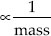As mass of car is smaller, therefore, acceleration of car is greater than the acceleration of the truck.
(D) The car is likely to suffer more damage than the truck, as it is lighter. The acceleration, i.e., change in velocity of car is more than that of the truck.